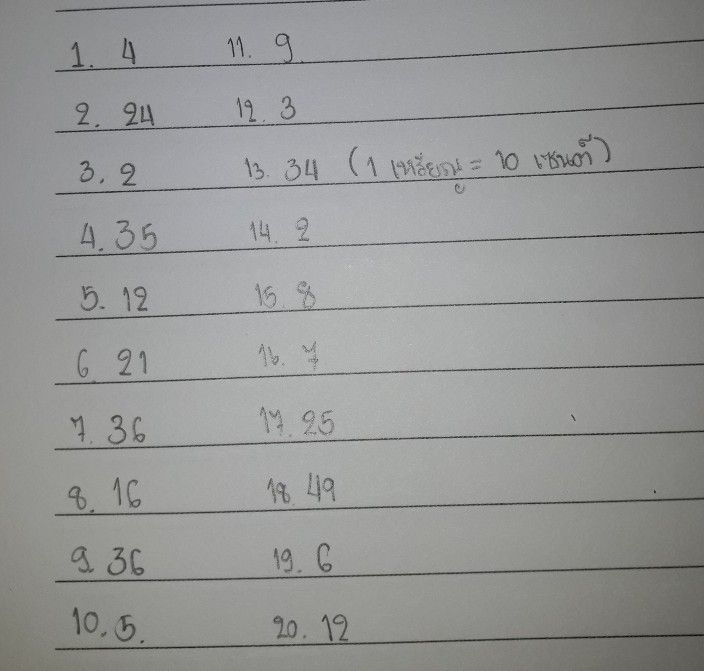เครื่องหมาย
โจทย์ปัญหาProportions and Rates Solve the following proportion equations 1 18 pounds to 22 pounds 2 12 pints $to36$ $pints$ 3 $16fee$ out of 18 feet 4 $25re$ bikes out $ot60bikes$ to 24 pennies 6 $9sn0wdaysowtot30days$ 5 12 pennies 7 $12b|ue$ cars out of 48 cars 8 32 quarts $to48$ quarts $9alons$ $to42$ gallons 10 $30bee$ $6ga1|$ beetles out of $35insects$ 12 $21^{.dimest024d}$ $simeS$ $12cuost021$ cups 14 $22C$ cakes out of 24 cakes 10 dimes out of 35 coins 16 21 inches to 28 inches 10 กickels to 18 nickeis 18 7 footballs $to56$ footballs 20 points out of 45 points 20 60 miles out of 72 miles 18 rainy days out of 24 days
มัธยมต้น
คณิตศาสตร์
วิธีการแก้ปัญหาคุณครู Qanda - Ammยังไม่เข้าใจใช่ไหม?
ถามคำถามกับครู Qanda